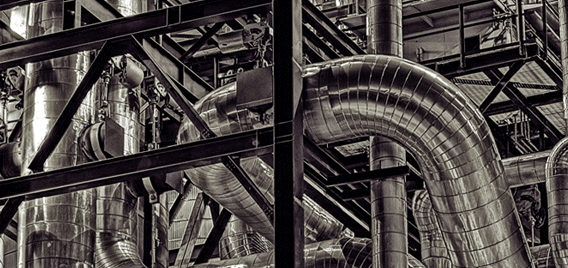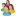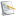### Chemical and Process Engineering Resources## Using Equivalent Lengths of Valves and Fittings

Nov 08 2010 01:20 PM | pleckner in Fluid FlowFinal Thoughts on K Values

The 1976 edition of the Crane Technical Paper No. 410 first discussed and used the two-friction factor method for calculating the total pressure drop in a piping system (f for straight pipe and ft for valves and fittings). Since then, Hooper2 suggested a 2-K method for calculating the pressure loss contribution for valves and fittings. His argument was that the equivalent length in pipe diameters (L/D) and K was indeed a function of Reynolds Number (at flow rates less than that obtained at fully developed turbulent flow) and the exact geometries of smaller valves and fittings. K for a given valve or fitting is a

combination of two Ks, one being the K found in CRANE Technical Paper No. 410, designated KY, and the other being defined as the K of the valve or fitting at a Reynolds Number equal to 1, designated K1. The two are related by the following equation:

K = K1 / NRE + K? (1 + 1/D)

The term (1+1/D) takes into account scaling between different sizes within a given valve or fitting group. Values for K1 can be found in the reference article2 and pressure drop is then calculated using Equation 7. For flow in the fully turbulent zone and larger size valves and fittings, K becomes consistent with that given in CRANE.

Darby3 expanded on the 2-K method. He suggests adding a third K term to the mix. Darby states that the 2-K method does not accurately represent the effect of scaling the sizes of valves and fittings. The reader is encouraged to get a copy of this article.

The use of the 2-K method has been around since 1981 and does not appear to have "caught" on as of yet. Some newer commercial computer programs allow for the use of the 2-K method, but most engineers inclined to use the K method instead of the Equivalent Length method still use the procedures given in CRANE. The latest 3-K method comes from data reported in the recent CCPS Guidlines4 and appears to be destined to become the new standard; we shall see.

Conclusion

Consistency, accuracy and correctness should be what the Process Design Engineer strives for. We all add our "fat" or safety factors to theoretical calculations to account for real-world situations. It would be comforting to know that the "fat" was added to a basis using sound and fundamentally correct methods for calculations.

### Fluid Flow Articleslidachang

yeal,thank you very much,i got a lot from  this topic.ksb
In Crane Tech Paper 410 (1982 version), Example 4-14 shows a 5"x4" Reducing Elbow which they model as a straight size 5" elbow and a sudden enlargement 4" to 5". In step 4 of Example 4-14, they calculate a pressure increase (negative pressure drop) according to the Bernoulli theorem, because the water slows down as it flows from the smaller diameter to the larger diameter. I was wondering why you didn't include the velocity change in your Sulfuric Acid example since it flows from the 1" pump discharge nozzle through the reducer to the 3" pipe. Since the velocity in the 1" nozzle is 31.22 ft/s, the Bernoulli dP would be -11.72 psi plus the frictional dP of 7.24 psi for a total pressure drop of -4.48 psi which is a pressure increase.I'm a bit confused by the preferred equations to be used. The only equation that produces the 11.73 psi dP for the system described is Equation 2, yet Equations 6 & 7 seem to be preferred.

•Industrial Forum

•Student Forum

•Refining Forum

•Simulation Forum

•Relief Devices Forum

•Tank Venting Forum

•Separation Technology

•Calculations and Tips

•Fluid Flow

•Heat Transfer

•Maintenance and Repair

•Utilities

•Safety and Pressure Relief

•Bulk Solids

•Process and Reactions

•Energy

•Other Topics

•For Students

•Physical Properties

•Archived Articles